# Homework 2: Desperately Seeking Silver (Solutions)¶

Due Thursday, Oct 3, 11:59 PMIn HW1, we explored how to make predictions (with uncertainties) about upcoming elections based on the Real Clear Politics poll. This assignment also focuses on election prediction, but we are going to implement and evaluate a number of more sophisticated forecasting techniques.

We are going to focus on the 2012 Presidential election. Analysts like Nate Silver, Drew Linzer, and Sam Wang developed highly accurate models that correctly forecasted most or all of the election outcomes in each of the 50 states. We will explore how hard it is to recreate similarly successful models. The goals of this assignment are:

1. To practice data manipulation with Pandas
2. To develop intuition about the interplay of precision, accuracy, and bias when making predictions
3. To better understand how election forecasts are constructed

The data for our analysis will come from demographic and polling data. We will simulate building our model on October 2, 2012 -- approximately one month before the election.

### Instructions¶

The questions in this assignment are numbered. The questions are also usually italicised, to help you find them in the flow of this notebook. At some points you will be asked to write functions to carry out certain tasks. Its worth reading a little ahead to see how the function whose body you will fill in will be used.

This is a long homework. Please do not wait until the last minute to start it!

The data for this homework can be found at this link. Download it to the same folder where you are running this notebook, and uncompress it. You should find the following files there:

1. us-states.json
3. predictwise.csv
4. g12.csv
5. g08.csv
6. 2008results.csv
7. nat.csv
8. p04.csv
9. 2012results.csv
10. cleaned-state_data2012.csv

#### Setup and Plotting code¶

In :
%matplotlib inline
from collections import defaultdict
import json

import numpy as np
import matplotlib.pyplot as plt
import pandas as pd

from matplotlib import rcParams
import matplotlib.cm as cm
import matplotlib as mpl

#colorbrewer2 Dark2 qualitative color table
dark2_colors = [(0.10588235294117647, 0.6196078431372549, 0.4666666666666667),
(0.8509803921568627, 0.37254901960784315, 0.00784313725490196),
(0.4588235294117647, 0.4392156862745098, 0.7019607843137254),
(0.9058823529411765, 0.1607843137254902, 0.5411764705882353),
(0.4, 0.6509803921568628, 0.11764705882352941),
(0.9019607843137255, 0.6705882352941176, 0.00784313725490196),
(0.6509803921568628, 0.4627450980392157, 0.11372549019607843)]

rcParams['figure.figsize'] = (10, 6)
rcParams['figure.dpi'] = 150
rcParams['axes.color_cycle'] = dark2_colors
rcParams['lines.linewidth'] = 2
rcParams['axes.facecolor'] = 'white'
rcParams['font.size'] = 14
rcParams['patch.edgecolor'] = 'white'
rcParams['patch.facecolor'] = dark2_colors
rcParams['font.family'] = 'StixGeneral'

def remove_border(axes=None, top=False, right=False, left=True, bottom=True):
"""
Minimize chartjunk by stripping out unnecesasry plot borders and axis ticks

The top/right/left/bottom keywords toggle whether the corresponding plot border is drawn
"""
ax = axes or plt.gca()
ax.spines['top'].set_visible(top)
ax.spines['right'].set_visible(right)
ax.spines['left'].set_visible(left)
ax.spines['bottom'].set_visible(bottom)

#turn off all ticks
ax.yaxis.set_ticks_position('none')
ax.xaxis.set_ticks_position('none')

#now re-enable visibles
if top:
ax.xaxis.tick_top()
if bottom:
ax.xaxis.tick_bottom()
if left:
ax.yaxis.tick_left()
if right:
ax.yaxis.tick_right()

pd.set_option('display.width', 500)
pd.set_option('display.max_columns', 100)

In :
#this mapping between states and abbreviations will come in handy later
states_abbrev = {
'AL': 'Alabama',
'AR': 'Arkansas',
'AS': 'American Samoa',
'AZ': 'Arizona',
'CA': 'California',
'CT': 'Connecticut',
'DC': 'District of Columbia',
'DE': 'Delaware',
'FL': 'Florida',
'GA': 'Georgia',
'GU': 'Guam',
'HI': 'Hawaii',
'IA': 'Iowa',
'ID': 'Idaho',
'IL': 'Illinois',
'IN': 'Indiana',
'KS': 'Kansas',
'KY': 'Kentucky',
'LA': 'Louisiana',
'MA': 'Massachusetts',
'MD': 'Maryland',
'ME': 'Maine',
'MI': 'Michigan',
'MN': 'Minnesota',
'MO': 'Missouri',
'MP': 'Northern Mariana Islands',
'MS': 'Mississippi',
'MT': 'Montana',
'NA': 'National',
'NC': 'North Carolina',
'ND': 'North Dakota',
'NH': 'New Hampshire',
'NJ': 'New Jersey',
'NM': 'New Mexico',
'NY': 'New York',
'OH': 'Ohio',
'OK': 'Oklahoma',
'OR': 'Oregon',
'PA': 'Pennsylvania',
'PR': 'Puerto Rico',
'RI': 'Rhode Island',
'SC': 'South Carolina',
'SD': 'South Dakota',
'TN': 'Tennessee',
'TX': 'Texas',
'UT': 'Utah',
'VA': 'Virginia',
'VI': 'Virgin Islands',
'VT': 'Vermont',
'WA': 'Washington',
'WI': 'Wisconsin',
'WV': 'West Virginia',
'WY': 'Wyoming'
}


Here is some code to plot State Chloropleth maps in matplotlib. make_map is the function you will use.

In :
#adapted from  https://github.com/dataiap/dataiap/blob/master/resources/util/map_util.py

state2poly = defaultdict(list)

for f in data['features']:
state = states_abbrev[f['id']]
geo = f['geometry']
if geo['type'] == 'Polygon':
for coords in geo['coordinates']:
state2poly[state].append(coords)
elif geo['type'] == 'MultiPolygon':
for polygon in geo['coordinates']:
state2poly[state].extend(polygon)

def draw_state(plot, stateid, **kwargs):
"""
draw_state(plot, stateid, color=..., **kwargs)

Automatically draws a filled shape representing the state in
subplot.
The color keyword argument specifies the fill color.  It accepts keyword
arguments that plot() accepts
"""
for polygon in state2poly[stateid]:
xs, ys = zip(*polygon)
plot.fill(xs, ys, **kwargs)

def make_map(states, label):
"""
Draw a cloropleth map, that maps data onto the United States

Inputs
-------
states : Column of a DataFrame
The value for each state, to display on a map
label : str
Label of the color bar

Returns
--------
The map
"""
fig = plt.figure(figsize=(12, 9))
ax = plt.gca()

if states.max() < 2: # colormap for election probabilities
cmap = cm.RdBu
vmin, vmax = 0, 1
else:  # colormap for electoral votes
cmap = cm.binary
vmin, vmax = 0, states.max()
norm = mpl.colors.Normalize(vmin=vmin, vmax=vmax)

skip = set(['National', 'District of Columbia', 'Guam', 'Puerto Rico',
'Virgin Islands', 'American Samoa', 'Northern Mariana Islands'])
for state in states_abbrev.values():
if state in skip:
continue
color = cmap(norm(states.ix[state]))
draw_state(ax, state, color = color, ec='k')

ax1 = fig.add_axes([0.45, 0.70, 0.4, 0.02])
cb1=mpl.colorbar.ColorbarBase(ax1, cmap=cmap,
norm=norm,
orientation='horizontal')
ax1.set_title(label)
remove_border(ax, left=False, bottom=False)
ax.set_xticks([])
ax.set_yticks([])
ax.set_xlim(-180, -60)
ax.set_ylim(15, 75)
return ax


## Today: the day we make the prediction¶

In :
# We are pretending to build our model 1 month before the election
import datetime
today = datetime.datetime(2012, 10, 2)
today

Out:
datetime.datetime(2012, 10, 2, 0, 0)

## Background: The Electoral College¶

US Presidential elections revolve around the Electoral College . In this system, each state receives a number of Electoral College votes depending on it's population -- there are 538 votes in total. In most states, all of the electoral college votes are awarded to the presidential candidate who recieves the most votes in that state. A candidate needs 269 votes to be elected President.

Thus, to calculate the total number of votes a candidate gets in the election, we add the electoral college votes in the states that he or she wins. (This is not entirely true, with Nebraska and Maine splitting their electoral college votes, but, for the purposes of this homework, we shall assume that the winner of the most votes in Maine and Nebraska gets ALL the electoral college votes there.)

Here is the electoral vote breakdown by state:

As a matter of convention, we will index all our dataframes by the state name

In :
electoral_votes = pd.read_csv("data/electoral_votes.csv").set_index('State')

Out:
State
California 55
Texas 38
New York 29
Florida 29
Illinois 20

To illustrate the use of make_map we plot the Electoral College

In :
make_map(electoral_votes.Votes, "Electoral Vlotes");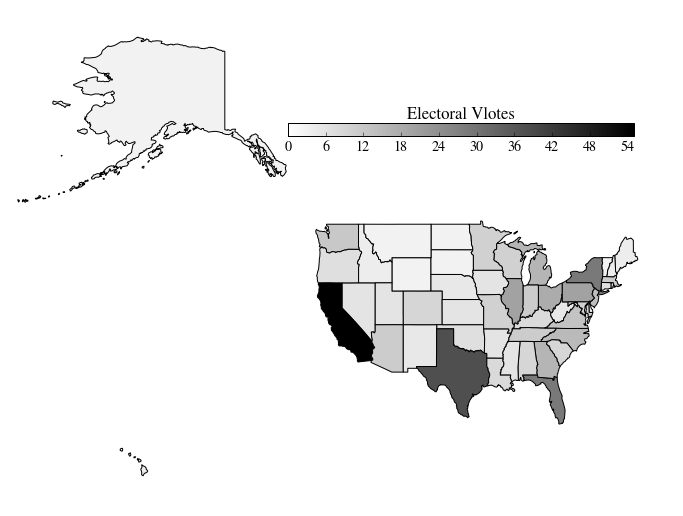## Question 1: Simulating elections¶

#### The PredictWise Baseline¶

We will start by examining a successful forecast that PredictWise made on October 2, 2012. This will give us a point of comparison for our own forecast models.

PredictWise aggregated polling data and, for each state, estimated the probability that the Obama or Romney would win. Here are those estimated probabilities:

In :
predictwise = pd.read_csv('data/predictwise.csv').set_index('States')

Out:
States
Alabama 0.000 1.000 9
Arizona 0.062 0.938 11
Arkansas 0.000 1.000 6
California 1.000 0.000 55

1.1 Each row is the probability predicted by Predictwise that Romney or Obama would win a state. The votes column lists the number of electoral college votes in that state. Use make_map to plot a map of the probability that Obama wins each state, according to this prediction.

In :
#your code here
make_map(predictwise.Obama, "P(Obama): PredictWise")

Out:
<matplotlib.axes.AxesSubplot at 0x108465090>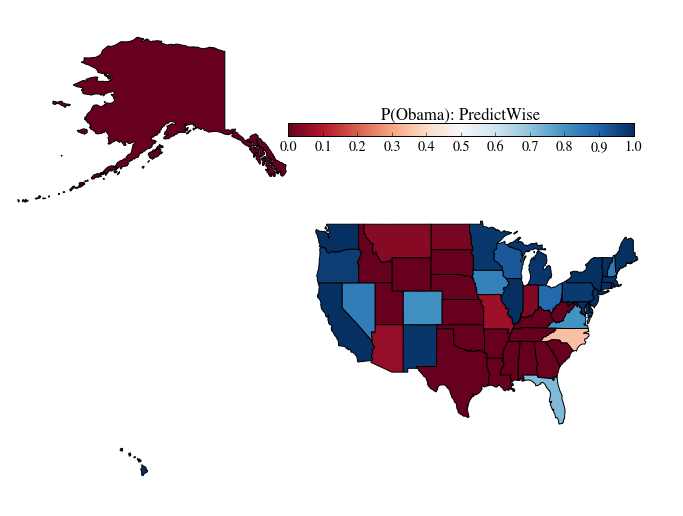Later on in this homework we will explore some approaches to estimating probabilities like these and quatifying our uncertainty about them. But for the time being, we will focus on how to make a prediction assuming these probabilities are known.

Even when we assume the win probabilities in each state are known, there is still uncertainty left in the election. We will use simulations from a simple probabilistic model to characterize this uncertainty. From these simulations, we will be able to make a prediction about the expected outcome of the election, and make a statement about how sure we are about it.

1.2 We will assume that the outcome in each state is the result of an independent coin flip whose probability of coming up Obama is given by a Dataframe of state-wise win probabilities. Write a function that uses this predictive model to simulate the outcome of the election given a Dataframe of probabilities.

In :
"""
Function
--------
simulate_election

Inputs
------
model : DataFrame
A DataFrame summarizing an election forecast. The dataframe has 51 rows -- one for each state and DC
It has the following columns:
Obama : Forecasted probability that Obama wins the state
The DataFrame is indexed by state (i.e., model.index is an array of state names)

n_sim : int
Number of simulations to run

Returns
-------
results : Numpy array with n_sim elements
Each element stores the number of electoral college votes Obama wins in each simulation.
"""

def simulate_election(model, n_sim):
#each column simulates a single outcome from the 50 states + DC
#Obama wins the simulation if the random number is < the win probability
simulations = np.random.uniform(size=(51, n_sim))
#summing over rows gives the total electoral votes for each simulation


The following cells takes the necessary DataFrame for the Predictwise data, and runs 10000 simulations. We use the results to compute the probability, according to this predictive model, that Obama wins the election (i.e., the probability that he receives 269 or more electoral college votes)

In :
result = simulate_election(predictwise, 10000)

In :
#compute the probability of an Obama win, given this simulation
print (result >= 269).mean()

0.9956


1.3 Now, write a function called plot_simulation to visualize the simulation. This function should:

• Build a histogram from the result of simulate_election
• Overplot the "victory threshold" of 269 votes as a vertical black line (hint: use axvline)
• Overplot the result (Obama winning 332 votes) as a vertical red line
• Compute the number of votes at the 5th and 95th quantiles, and display the difference (this is an estimate of the outcome's uncertainty)
• Display the probability of an Obama victory
In :
"""
Function
--------
plot_simulation

Inputs
------
simulation: Numpy array with n_sim (see simulate_election) elements
Each element stores the number of electoral college votes Obama wins in each simulation.

Returns
-------
Nothing
"""

def plot_simulation(simulation):
plt.hist(simulation, bins=np.arange(200, 538, 1),
label='simulations', align='left', normed=True)
plt.axvline(332, 0, .5, color='r', label='Actual Outcome')
plt.axvline(269, 0, .5, color='k', label='Victory Threshold')
p05 = np.percentile(simulation, 5.)
p95 = np.percentile(simulation, 95.)
iq = int(p95 - p05)
pwin = ((simulation >= 269).mean() * 100)
plt.legend(frameon=False, loc='upper left')
plt.ylabel("Probability")
remove_border()


Lets plot the result of the Predictwise simulation. Your plot should look something like this:In :
plot_simulation(result)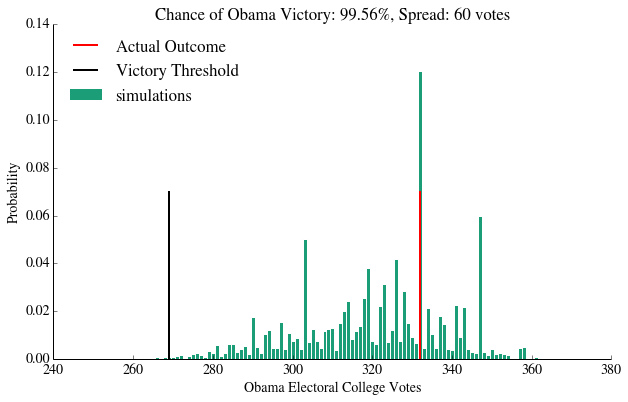#### Evaluating and Validating our Forecast¶

The point of creating a probabilistic predictive model is to simultaneously make a forecast and give an estimate of how certain we are about it.

However, in order to trust our prediction or our reported level of uncertainty, the model needs to be correct. We say a model is correct if it honestly accounts for all of the mechanisms of variation in the system we're forecasting.

In this section, we evaluate our prediction to get a sense of how useful it is, and we validate the predictive model by comparing it to real data.

1.4 Suppose that we believe the model is correct. Under this assumption, we can evaluate our prediction by characterizing its accuracy and precision (see here for an illustration of these ideas). What does the above plot reveal about the accuracy and precision of the PredictWise model?

Background: To make a prediction, we take information that we have now, and try to identify likely outcomes in the future based on this information. The model we've created expresses our uncertainty as a probability distribution over the likely outcomes of the election that are consistent with the information we have now. We call this distribution over outcomes the predictive distribution. Simulating from this model and plotting a histogram allows us to visualize the predictive distribution. When we say a model is correct, we mean that the predictive distribution matches the true distribution of election outcomes when the information leading up to the election matches the information that we have now.

Usually, people are interested in having a single value as the prediction ("Obama will get 332 votes"). To obtain a prediction, we summarize the predictive distribution with a single point, usually by taking its expectation. We can evaluate a prediction by its accuracy and precision.

Answer: To evaluate the accuracy of our prediction, we can check to see whether the expectation of our predictive distribution seems to match the expectation of the true outcome. In this case, much of the predictive distribution's mass lies on or around the real outcome (that is, the histogram is approximately centered on the actual outcome of Obama=332 votes). So, based on the outcome we observed, the model seems accurate. To make a more rigorous statement about accuracy, we would want to have more replications (that is, more elections) to see whether the expectation of the predictive distribution consistently matches the true outcomes.

To evaluate the precision of our prediction, we look at the spread of the histogram. Because we are assuming the model is correct, we can interpret the spread of the histogram as a measure of the variability among the election outcomes that are consistent with the information we have. If our current information does not constrain the likely election outcomes very much, then the difference between our prediction and the true outcome can vary widely. The spread of the histogram is 60 votes, which is relatively large. Whether the prediction is precise enough is a question of what you want to do with your prediction. For example, if you want to be able to call the winner of a close election (say the candidates are separated by less than 30 votes), this prediction would not be precise enough to identify a winner with 95% confidence. To handle this, we might wish to incorporate more information into the model to reduce the spread of likely election outcomes.

1.5 Unfortunately, we can never be absolutely sure that a model is correct, just as we can never be absolutely sure that the sun will rise tomorrow. But we can test a model by making predictions assuming that it is true and comparing it to real events -- this constitutes a hypothesis test. After testing a large number of predictions, if we find no evidence that says the model is wrong, we can have some degree of confidence that the model is right (the same reason we're still quite confident about the sun being here tomorrow). We call this process model checking, and use it to validate our model.

Describe how the graph provides one way of checking whether the prediction model is correct. How many predictions have we checked in this case? How could we increase our confidence in the model's correctness?

Answer: The graph shows a hypothesis test. The histogram approximates the predictive distribution of election outcomes (in terms of electoral votes) assuming our model for the election is true. By comparing this to the true outcome of the election, we can see whether the observed electoral vote count would be highly atypical if the model were true. In this case, it appears the true outcome is quite typical among the model's predicted outcomes, so we do not reject our model.

In this case, we have checked only one prediction, since there is only one true outcome that we've compared to the predictive distribution.

To increase our confidence, we would want to test more outcomes against predictions that were made in the same way. For example, we could apply the same procedure (including PredictWise's computation of the statewise probability estimates) to different elections and see whether each of the hypothesis tests fails to reject the model in those cases as well. We could also break the election down into state-by-state outcomes, and test the prediction for each state against that state's outcome.

### Gallup Party Affiliation Poll¶

Now we will try to estimate our own win probabilities to plug into our predictive model.

We will start with a simple forecast model. We will try to predict the outcome of the election based the estimated proportion of people in each state who identify with one one political party or the other.

Gallup measures the political leaning of each state, based on asking random people which party they identify or affiliate with. Here's the data they collected from January-June of 2012:

In :
gallup_2012=pd.read_csv("data/g12.csv").set_index('State')
gallup_2012["Unknown"] = 100 - gallup_2012.Democrat - gallup_2012.Republican

Out:
State
Alabama 36.0 49.6 -13.6 3197 14.4
Alaska 35.9 44.3 -8.4 402 19.8
Arizona 39.8 47.3 -7.5 4325 12.9
Arkansas 41.5 40.8 0.7 2071 17.7
California 48.3 34.6 13.7 16197 17.1

Each row lists a state, the percent of surveyed individuals who identify as Democrat/Republican, the percent whose identification is unknown or who haven't made an affiliation yet, the margin between Democrats and Republicans (Dem_Adv: the percentage identifying as Democrats minus the percentage identifying as Republicans), and the number N of people surveyed.

1.6 This survey can be used to predict the outcome of each State's election. The simplest forecast model assigns 100% probability that the state will vote for the majority party. Implement this simple forecast.

In :
"""
Function
--------
simple_gallup_model

A simple forecast that predicts an Obama (Democratic) victory with
0 or 100% probability, depending on whether a state
leans Republican or Democrat.

Inputs
------
gallup : DataFrame
The Gallup dataframe above

Returns
-------
model : DataFrame
A dataframe with the following column
* Obama: probability that the state votes for Obama. All values should be 0 or 1
model.index should be set to gallup.index (that is, it should be indexed by state name)

Examples
---------
>>> simple_gallup_model(gallup_2012).ix['Florida']
Obama    1
Name: Florida, dtype: float64
>>> simple_gallup_model(gallup_2012).ix['Arizona']
Obama    0
Name: Arizona, dtype: float64
"""

def simple_gallup_model(gallup):


Now, we run the simulation with this model, and plot it.

In :
model = simple_gallup_model(gallup_2012)
prediction = simulate_election(model, 10000)

plot_simulation(prediction)
plt.show()
make_map(model.Obama, "P(Obama): Simple Model")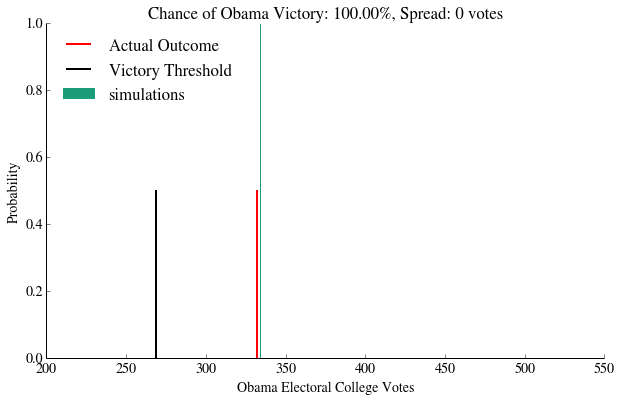Out:
<matplotlib.axes.AxesSubplot at 0x108d3ffd0>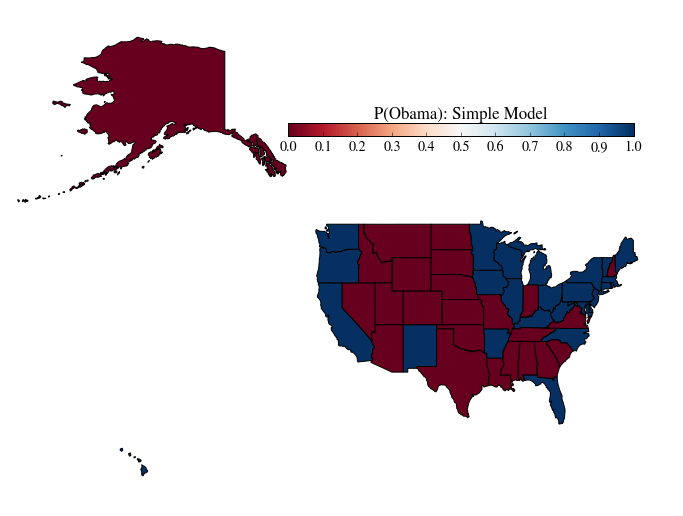1.7 Attempt to validate the predictive model using the above simulation histogram. Does the evidence contradict the predictive model?

Answer: We do not predict the exactly correct result (red line). According to the predictive model, the true outcome has probability 0. Thus, the evidence contradicts the predictive model, and we should reject it.

#### Adding Polling Uncertainty to the Predictive Model¶

The model above is brittle -- it includes no accounting for uncertainty, and thus makes predictions with 100% confidence. This is clearly wrong -- there are numerous sources of uncertainty in estimating election outcomes from a poll of affiliations.

The most obvious source of error in the Gallup data is the finite sample size -- Gallup did not poll everybody in America, and thus the party affilitions are subject to sampling errors. How much uncertainty does this introduce?

On their webpage discussing these data, Gallup notes that the sampling error for the states is between 3 and 6%, with it being 3% for most states. (The calculation of the sampling error itself is an exercise in statistics. Its fun to think of how you could arrive at the sampling error if it was not given to you. One way to do it would be to assume this was a two-choice situation and use binomial sampling error for the non-unknown answers, and further model the error for those who answered 'Unknown'.)

1.8 Use Gallup's estimate of 3% to build a Gallup model with some uncertainty. Assume that the Dem_Adv column represents the mean of a Gaussian, whose standard deviation is 3%. Build the model in the function uncertain_gallup_model. Return a forecast where the probability of an Obama victory is given by the probability that a sample from the Dem_Adv Gaussian is positive.

Hint The probability that a sample from a Gaussian with mean $\mu$ and standard deviation $\sigma$ exceeds a threhold $z$ can be found using the the Cumulative Distribution Function of a Gaussian:

$$CDF(z) = \frac1{2}\left(1 + {\rm erf}\left(\frac{z - \mu}{\sqrt{2 \sigma^2}}\right)\right)$$
In :
"""
Function
--------
uncertain_gallup_model

A forecast that predicts an Obama (Democratic) victory if the random variable drawn
from a Gaussian with mean Dem_Adv and standard deviation 3% is >0

Inputs
------
gallup : DataFrame
The Gallup dataframe above

Returns
-------
model : DataFrame
A dataframe with the following column
* Obama: probability that the state votes for Obama.
model.index should be set to gallup.index (that is, it should be indexed by state name)
"""
from scipy.special import erf
def uncertain_gallup_model(gallup):
sigma = 3
prob =  .5 * (1 + erf(gallup.Dem_Adv / np.sqrt(2 * sigma**2)))
return pd.DataFrame(dict(Obama=prob), index=gallup.index)


We construct the model by estimating the probabilities:

In :
model = uncertain_gallup_model(gallup_2012)


Once again, we plot a map of these probabilities, run the simulation, and display the results

In :
make_map(model.Obama, "P(Obama): Gallup + Uncertainty")
plt.show()
prediction = simulate_election(model, 10000)
plot_simulation(prediction)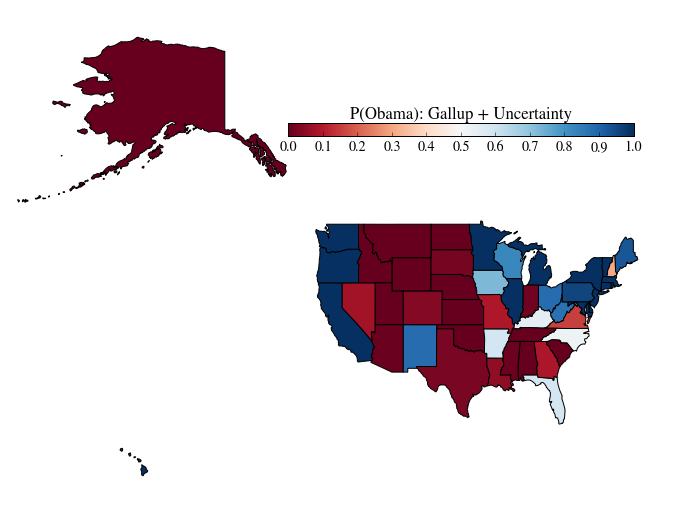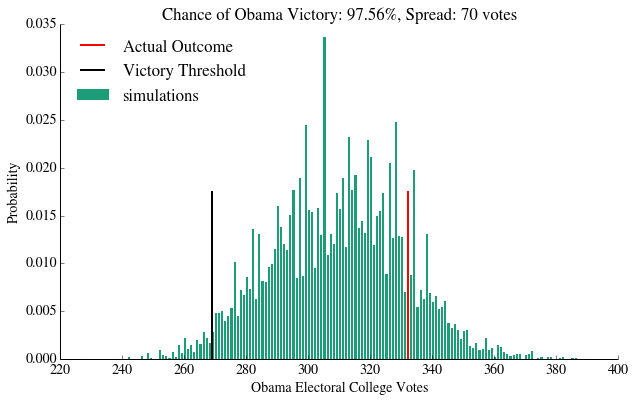1.9 Attempt to validate the above model using the histogram. Does the predictive distribution appear to be consistent with the real data? Comment on the accuracy and precision of the prediction.

Answer: The predictive distribution is consistent with the real data -- the real outcome seems like a typical outcome according to the model. The accuracy is not very good as the center of the distribution falls fairly far from the observed outcome, but the precision is only marginally worse than in the predictwise case.

#### Biases¶

While accounting for uncertainty is one important part of making predictions, we also want to avoid systematic errors. We call systematic over- or under-estimation of an unknown quantity bias. In the case of this forecast, our predictions would be biased if the estimates from this poll systematically over- or under-estimate vote proportions on election day. There are several reasons this might happen:

1. Gallup is wrong. The poll may systematically over- or under-estimate party affiliation. This could happen if the people who answer Gallup phone interviews might not be a representative sample of people who actually vote, Gallup's methodology is flawed, or if people lie during a Gallup poll.
2. Our assumption about party affiliation is wrong. Party affiliation may systematically over- or under-estimate vote proportions. This could happen if people identify with one party, but strongly prefer the candidate from the other party, or if undecided voters do not end up splitting evenly between Democrats and Republicans on election day.
3. Our assumption about equilibrium is wrong. This poll was released in August, with more than two months left for the elections. If there is a trend in the way people change their affiliations during this time period (for example, because one candidate is much worse at televised debates), an estimate in August could systematically miss the true value in November.

One way to account for bias is to calibrate our model by estimating the bias and adjusting for it. Before we do this, let's explore how sensitive our prediction is to bias.

1.10 Implement a biased_gallup forecast, which assumes the vote share for the Democrat on election day will be equal to Dem_Adv shifted by a fixed negative amount. We will call this shift the "bias", so a bias of 1% means that the expected vote share on election day is Dem_Adv-1.

Hint You can do this by wrapping the uncertain_gallup_model in a function that modifies its inputs.

In :
"""
Function
--------
biased_gallup_poll

Subtracts a fixed amount from Dem_Adv, beofore computing the uncertain_gallup_model.
This simulates correcting a hypothetical bias towards Democrats
in the original Gallup data.

Inputs
-------
gallup : DataFrame
The Gallup party affiliation data frame above
bias : float
The amount by which to shift each prediction

Examples
--------
>>> model = biased_gallup(gallup, 1.)
>>> model.ix['Flordia']
>>> .460172
"""
def biased_gallup(gallup, bias):
g2 = gallup.copy()
return uncertain_gallup_model(g2)


1.11 Simulate elections assuming a bias of 1% and 5%, and plot histograms for each one.

In :
#your code here
model = biased_gallup(gallup_2012, 1)
prediction = simulate_election(model, 10000)
plot_simulation(prediction)
plt.show()

model = biased_gallup(gallup_2012, 5)
prediction = simulate_election(model, 10000)
plot_simulation(prediction)
plt.show()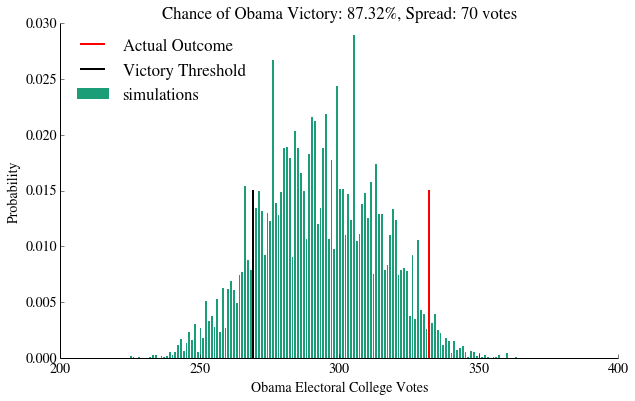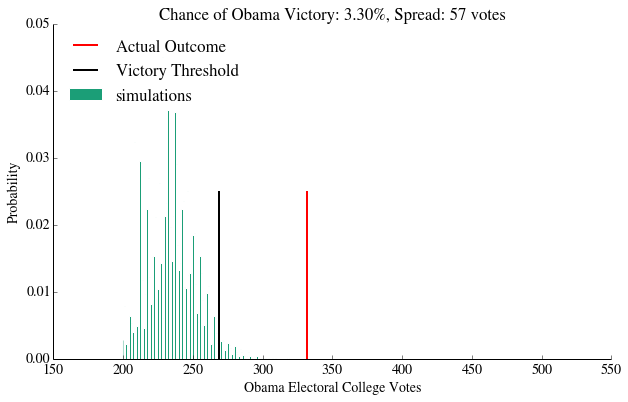Note that even a small bias can have a dramatic effect on the predictions. Pundits made a big fuss about bias during the last election, and for good reason -- it's an important effect, and the models are clearly sensitive to it. Forecastors like Nate Silver would have had an easier time convincing a wide audience about their methodology if bias wasn't an issue.

Furthermore, because of the nature of the electoral college, biases get blown up large. For example, suppose you mis-predict the party Florida elects. We've possibly done this as a nation in the past :-). Thats 29 votes right there. So, the penalty for even one misprediction is high.

#### Estimating the size of the bias from the 2008 election¶

While bias can lead to serious inaccuracy in our predictions, it is fairly easy to correct if we are able to estimate the size of the bias and adjust for it. This is one form of calibration.

One approach to calibrating a model is to use historical data to estimate the bias of a prediction model. We can use our same prediction model on historical data and compare our historical predictions to what actually occurred and see if, on average, the predictions missed the truth by a certain amount. Under some assumptions (discussed in a question below), we can use the estimate of the bias to adjust our current forecast.

In this case, we can use data from the 2008 election. (The Gallup data from 2008 are from the whole of 2008, including after the election):

In :
gallup_08 = pd.read_csv("data/g08.csv").set_index('State')

prediction_08['Dem_Win']=results_08["Obama Pct"] - results_08["McCain Pct"]

Out:
State
Alabama -0.8 -21.58
Arizona -0.4 -8.52
Arkansas 12.5 -19.86
California 19.4 24.06

1.12 Make a scatter plot using the prediction_08 dataframe of the democratic advantage in the 2008 Gallup poll (X axis) compared to the democratic win percentage -- the difference between Obama and McCain's vote percentage -- in the election (Y Axis). Overplot a linear fit to these data.

Hint The np.polyfit function can compute linear fits, as can sklearn.linear_model.LinearModel

In :
#your code here

plt.ylabel("2008 Election Democrat Win")
x = np.linspace(-40, 80, 10)
y = np.polyval(fit, x)
plt.plot(x, y)
print fit

[  1.26390486 -11.32855786]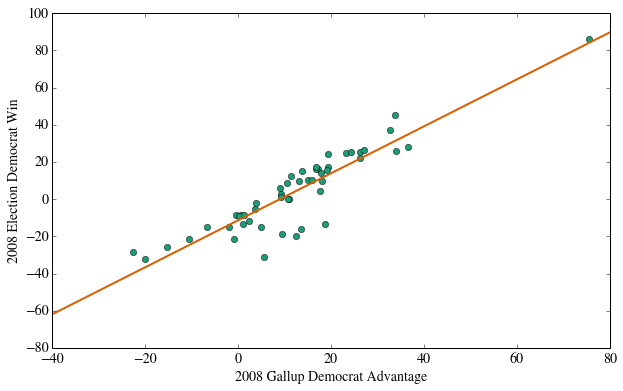Notice that a lot of states in which Gallup reported a Democratic affiliation, the results were strongly in the opposite direction. Why might that be? You can read more about the reasons for this here.

A quick look at the graph will show you a number of states where Gallup showed a Democratic advantage, but where the elections were lost by the democrats. Use Pandas to list these states.

In :
#your code here
prediction_08[(prediction_08.Dem_Win < 0) & (prediction_08.Dem_Adv > 0)]

Out:
State
Arkansas 12.5 -19.86
Georgia 3.6 -5.21
Kentucky 13.5 -16.23
Louisiana 9.4 -18.63
Mississippi 1.1 -13.18
Missouri 10.9 -0.14
Montana 3.9 -2.26
North Dakota 0.6 -8.63
Oklahoma 5.6 -31.30
South Carolina 0.1 -8.97
South Dakota 1.3 -8.41
Tennessee 5.0 -15.07
Texas 2.4 -11.77
West Virginia 18.8 -13.12

We compute the average difference between the Democrat advantages in the election and Gallup poll

In :
print (prediction_08.Dem_Adv - prediction_08.Dem_Win).mean()

8.06803921569


Answer The bias was roughly 8% in favor of the Democrats in the Gallup Poll, meaning that you would want to adjust predictions based on this poll down by that amount. This was the result of people in a number of Southern and Western states claiming to be affiliated as Democrats, then voting the other way. Or, since Gallup kept polling even after the elections, it could also represent people swept away by the 2008 election euphoria in those states. This is an illustration of why one needs to be carefull with polls.

1.13 Calibrate your forecast of the 2012 election using the estimated bias from 2008. Validate the resulting model against the real 2012 outcome. Did the calibration help or hurt your prediction?

In :
#your code here
model = biased_gallup(gallup_2012, 8.06)
prediction = simulate_election(model, 10000)
plot_simulation(prediction)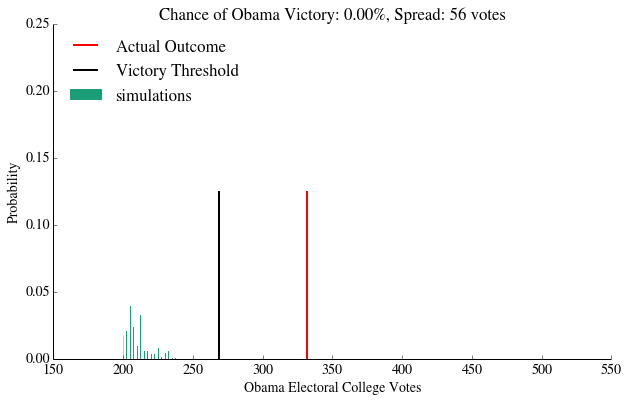Answer: This was a disaster. The 8% calibration completey destroys the accuracy of our prediction in 2012. Our calibration made the assumptions that a) the bias in 2008 was the same as 2012, and b) the bias in each state was the same.

There are several ways in which these assumptions may have been violated. Gallup may have changed their methodology to account for this bias already, leading to a different bias in 2012 from what there was in 2008. The state-by-state biases may have also been different -- voters in highly conservative states may have responded to polls differently from voters in libreral states, for instance. It might have been better to callibrate the bias on a state-wide or clustered basis.

1.14 Finally, given that we know the actual outcome of the 2012 race, and what you saw from the 2008 race would you trust the results of the an election forecast based on the 2012 Gallup party affiliation poll?

Answer: No. You should answer this question as though you had not yet seen the results of the 2012 election. The results from 2008 would suggest that the party affiliation poll is a highly biased predictor of the acutal election outcome. Given that calibrating the model to counteract this bias would rely on unrealistic assumptions, it would seem unwise to use the 2012 party affiliation poll to predict the election.

## Question 2: Logistic Considerations¶

In the previous forecast, we used the strategy of taking some side-information about an election (the partisan affiliation poll) and relating that to the predicted outcome of the election. We tied these two quantities together using a very simplistic assumption, namely that the vote outcome is deterministically related to estimated partisan affiliation.

In this section, we use a more sophisticated approach to link side information -- usually called features or predictors -- to our prediction. This approach has several advantages, including the fact that we may use multiple features to perform our predictions. Such data may include demographic data, exit poll data, and data from previous elections.

First, we'll construct a new feature called PVI, and use it and the Gallup poll to build predictions. Then, we'll use logistic regression to estimate win probabilities, and use these probabilities to build a prediction.

#### The Partisan Voting Index¶

The Partisan Voting Index (PVI) is defined as the excessive swing towards a party in the previous election in a given state. In other words:

$$PVI_{2008} (state) = Democratic.Percent_{2004} ( state ) - Republican.Percent_{2004} ( state) - \\ \Big ( Democratic.Percent_{2004} (national) - Republican.Percent_{2004} (national) \Big )$$

To calculate it, let us first load the national percent results for republicans and democrats in the last 3 elections and convert it to the usual democratic - republican format.

In :
national_results=pd.read_csv("data/nat.csv")
national_results.set_index('Year',inplace=True)

Out:
Dem Rep
Year
2004 48 51
2008 53 46
2012 51 47

Let us also load in data about the 2004 elections from p04.csv which gets the results in the above form for the 2004 election for each state.

In :
polls04=pd.read_csv("data/p04.csv")
polls04.State=polls04.State.replace(states_abbrev)
polls04.set_index("State", inplace=True);

Out:
Dem Rep
State
Alabama 37 63
Arizona 44 55
Arkansas 45 54
California 54 45
In :
pvi08=polls04.Dem - polls04.Rep - (national_results.xs(2004)['Dem'] - national_results.xs(2004)['Rep'])

Out:
State
Alabama      -23
Arizona       -8
Arkansas      -6
California    12
dtype: int64

2.1 Build a new DataFrame called e2008. The dataframe e2008 must have the following columns:

• a column named pvi with the contents of the partisan vote index pvi08
• a column named Dem_Adv which has the Democratic advantage from the frame prediction_08 of the last question with the mean subtracted out
• a column named obama_win which has a 1 for each state Obama won in 2008, and 0 otherwise
• a column named Dem_Win which has the 2008 election Obama percentage minus McCain percentage, also from the frame prediction_08
• The DataFrame should be indexed and sorted by State
In :
#your code here
e2008['obama_win']=1*(prediction_08.Dem_Win > 0)
e2008 = e2008.sort_index()

Out:
State
Alabama -13.154902 -21.58 -23 0
Arizona -12.754902 -8.52 -8 0
Arkansas 0.145098 -19.86 -6 0
California 7.045098 24.06 12 1

We construct a similar frame for 2012, obtaining pvi using the 2008 Obama win data which we already have. There is no obama_win column since, well, our job is to predict it!

In :
pvi12 = e2008.Dem_Win - (national_results.xs(2008)['Dem'] - national_results.xs(2008)['Rep'])
e2012 = e2012.sort_index()

Out:
State
Alabama -14.684314 -28.58
Arizona -8.584314 -15.52
Arkansas -0.384314 -26.86
California 12.615686 17.06

We load in the actual 2012 results so that we can compare our results to the predictions.

In :
results2012 = pd.read_csv("data/2012results.csv")
results2012.set_index("State", inplace=True)
results2012 = results2012.sort_index()

Out:
Winner
State
Alabama 0
Arizona 0
Arkansas 0
California 1

#### Exploratory Data Analysis¶

2.2 Lets do a little exploratory data analysis. Plot a scatter plot of the two PVi's against each other. What are your findings? Is the partisan vote index relatively stable from election to election?

In :
#your code here
plt.plot(e2008.pvi, e2012.pvi, 'o', label='Data')
fit = np.polyfit(e2008.pvi, e2012.pvi, 1)
x = np.linspace(-40, 80, 10)
y = np.polyval(fit, x)
plt.plot(x, x, '--k', alpha=.3, label='x=y')
plt.plot(x, y, label='Linear fit')
plt.xlabel("2004 PVI")
plt.ylabel("2008 PVI")
plt.legend(loc='upper left')

Out:
<matplotlib.legend.Legend at 0x109e19b10>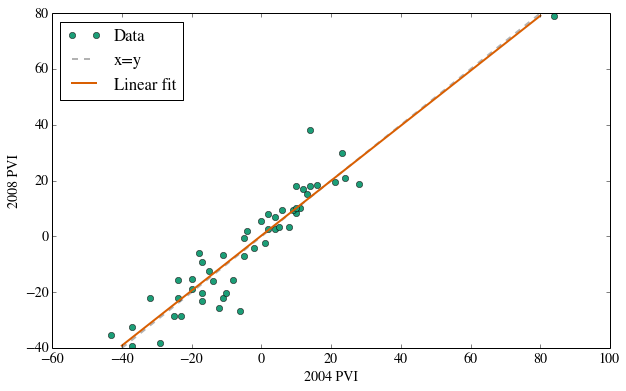Answer: There is a reasonably well fit line which hugs the x=y line. This tells us that the PVI seems relatively stable from election to election and may thus make a good predictor.

2.3 Lets do a bit more exploratory data analysis. Using a scatter plot, plot Dem_Adv against pvi in both 2008 and 2012. Use colors red and blue depending upon obama_win for the 2008 data points. Plot the 2012 data using gray color. Is there the possibility of making a linear separation (line of separation) between the red and the blue points on the graph?

In :
#your code here

plt.ylabel("pvi")
colors=["red","blue"]
ax=plt.gca()
for label in [0, 1]:
color = colors[label]
l = '2008 McCain States' if label == 0 else '2008 Obama States'

marker="s", label='2012 States', alpha=.3)
plt.legend(frameon=False, scatterpoints=1, loc='upper left')
remove_border()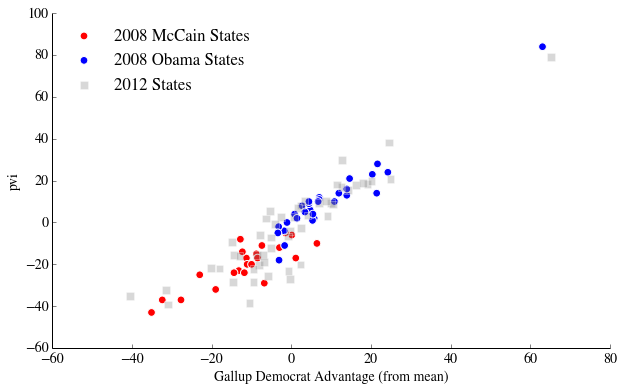Answer: Yes, it seems that with 3-4 misclassifications, we could draw a line to divide the data into two parts.

#### The Logistic Regression¶

Logistic regression is a probabilistic model that links observed binary data to a set of features.

Suppose that we have a set of binary (that is, taking the values 0 or 1) observations $Y_1,\cdots,Y_n$, and for each observation $Y_i$ we have a vector of features $X_i$. The logistic regression model assumes that there is some set of weights, coefficients, or parameters $\beta$, one for each feature, so that the data were generated by flipping a weighted coin whose probability of giving a 1 is given by the following equation:

$$P(Y_i = 1) = \mathrm{logistic}(\sum \beta_i X_i),$$

where

$$\mathrm{logistic}(x) = \frac{e^x}{1+e^x}.$$

When we fit a logistic regression model, we determine values for each $\beta$ that allows the model to best fit the training data we have observed (the 2008 election). Once we do this, we can use these coefficients to make predictions about data we have not yet observed (the 2012 election).

Sometimes this estimation procedure will overfit the training data yielding predictions that are difficult to generalize to unobserved data. Usually, this occurs when the magnitudes of the components of $\beta$ become too large. To prevent this, we can use a technique called regularization to make the procedure prefer parameter vectors that have smaller magnitude. We can adjust the strength of this regularization to reduce the error in our predictions.

We now write some code as technology for doing logistic regression. By the time you start doing this homework, you will have learnt the basics of logistic regression, but not all the mechanisms of cross-validation of data sets. Thus we provide here the code for you to do the logistic regression, and the accompanying cross-validation.

We first build the features from the 2008 data frame, returning y, the vector of labels, and X the feature-sample matrix where the columns are the features in order from the list featurelist, and each row is a data "point".

In :
from sklearn.linear_model import LogisticRegression

def prepare_features(frame2008, featureslist):
y= frame2008.obama_win.values
X = frame2008[featureslist].values
if len(X.shape) == 1:
X = X.reshape(-1, 1)
return y, X


We use the above function to get the label vector and feature-sample matrix for feeding to scikit-learn. We then use the usual scikit-learn incantation fit to fit a logistic regression model with regularization parameter C. The parameter C is a hyperparameter of the model, and is used to penalize too high values of the parameter co-efficients in the loss function that is minimized to perform the logistic regression. We build a new dataframe with the usual Obama column, that holds the probabilities used to make the prediction. Finally we return a tuple of the dataframe and the classifier instance, in that order.

In :
def fit_logistic(frame2008, frame2012, featureslist, reg=0.0001):
y, X = prepare_features(frame2008, featureslist)
clf2 = LogisticRegression(C=reg)
clf2.fit(X, y)
X_new = frame2012[featureslist]
obama_probs = clf2.predict_proba(X_new)[:, 1]

df = pd.DataFrame(index=frame2012.index)
df['Obama'] = obama_probs
return df, clf2


We are not done yet. In order to estimate C, we perform a grid search over many C to find the best C that minimizes the loss function. For each point on that grid, we carry out a n_folds-fold cross-validation. What does this mean?

Suppose n_folds=10. Then we will repeat the fit 10 times, each time randomly choosing 50/10 ~ 5 states out as a test set, and using the remaining 45/46 as the training set. We use the average score on the test set to score each particular choice of C, and choose the one with the best performance.

In :
from sklearn.grid_search import GridSearchCV

def cv_optimize(frame2008, featureslist, n_folds=10, num_p=100):
y, X = prepare_features(frame2008, featureslist)
clf = LogisticRegression()
parameters = {"C": np.logspace(-4, 3, num=num_p)}
gs = GridSearchCV(clf, param_grid=parameters, cv=n_folds)
gs.fit(X, y)
return gs.best_params_, gs.best_score_


Finally we write the function that we use to make our fits. It takes both the 2008 and 2012 frame as arguments, as well as the featurelist, and the number of cross-validation folds to do. It uses the above defined logistic_score to find the best-fit C, and then uses this value to return the tuple of result dataframe and classifier described above. This is the function you will be using.

In :
def cv_and_fit(frame2008, frame2012, featureslist, n_folds=5):
bp, bs = cv_optimize(frame2008, featureslist, n_folds=n_folds)
predict, clf = fit_logistic(frame2008, frame2012, featureslist, reg=bp['C'])
return predict, clf


2.4 *Carry out a logistic fit using the cv_and_fit function developed above. As your featurelist use the features we have: Dem_Adv and pvi.

In :
#your code here
res, clf = cv_and_fit(e2008, e2012, ['Dem_Adv', 'pvi'])

Out:
State
Alabama 0.004234 9
Arizona 0.069288 11
Arkansas 0.031901 6
California 0.994956 55

2.5 As before, plot a histogram and map of the simulation results, and interpret the results in terms of accuracy and precision.

In :
#code to make the histogram

prediction = simulate_election(predict2012_logistic, 10000)
plot_simulation(prediction)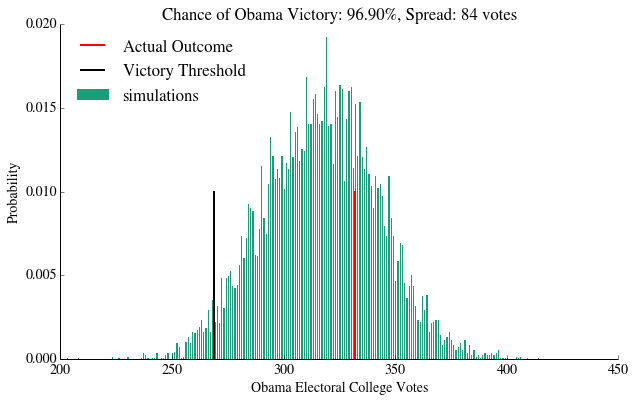In :
#code to make the map

make_map(predict2012_logistic.Obama, "P(Obama): Logistic")

Out:
<matplotlib.axes.AxesSubplot at 0x10c274890>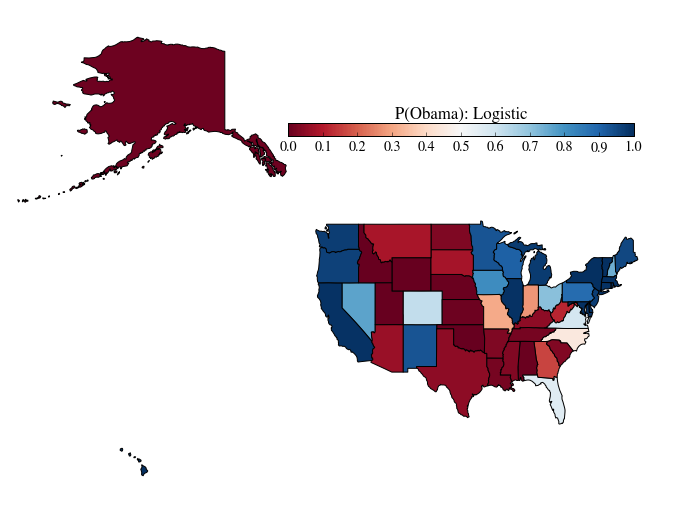Answer: The precision has worsened with respect to the predictwise (and even the gallup) model. The accuracy has improved with respect to the gallup model, but is not as good as in the predictwise model.

#### Classifier Decision boundary¶

One nice way to visualize a 2-dimensional logistic regression is to plot the probability as a function of each dimension. This shows the decision boundary -- the set of parameter values where the logistic fit yields P=0.5, and shifts between a preference for Obama or McCain/Romney.

The function below draws such a figure (it is adapted from the scikit-learn website), and overplots the data.

In :
from matplotlib.colors import ListedColormap
def points_plot(e2008, e2012, clf):
"""
e2008: The e2008 data
e2012: The e2012 data
clf: classifier
"""
ytrain = e2008['obama_win'].values == 1

X=np.concatenate((Xtrain, Xtest))

# evenly sampled points
x_min, x_max = X[:, 0].min() - .5, X[:, 0].max() + .5
y_min, y_max = X[:, 1].min() - .5, X[:, 1].max() + .5
xx, yy = np.meshgrid(np.linspace(x_min, x_max, 50),
np.linspace(y_min, y_max, 50))
plt.xlim(xx.min(), xx.max())
plt.ylim(yy.min(), yy.max())

#plot background colors
ax = plt.gca()
Z = clf.predict_proba(np.c_[xx.ravel(), yy.ravel()])[:, 1]
Z = Z.reshape(xx.shape)
cs = ax.contourf(xx, yy, Z, cmap='RdBu', alpha=.5)
cs2 = ax.contour(xx, yy, Z, cmap='RdBu', alpha=.5)
plt.clabel(cs2, fmt = '%2.1f', colors = 'k', fontsize=14)

# Plot the 2008 points
ax.plot(Xtrain[ytrain == 0, 0], Xtrain[ytrain == 0, 1], 'ro', label='2008 McCain')
ax.plot(Xtrain[ytrain == 1, 0], Xtrain[ytrain == 1, 1], 'bo', label='2008 Obama')

# and the 2012 points
ax.scatter(Xtest[:, 0], Xtest[:, 1], c='k', marker="s", s=50, facecolors="k", alpha=.5, label='2012')
plt.legend(loc='upper left', scatterpoints=1, numpoints=1)

return ax


2.6 Plot your results on the classification space boundary plot. How sharp is the classification boundary, and how does this translate into accuracy and precision of the results?

In :
#your code here
points_plot(e2008, e2012, clf)
plt.ylabel("PVI")

Out:
<matplotlib.text.Text at 0x10da5cc90>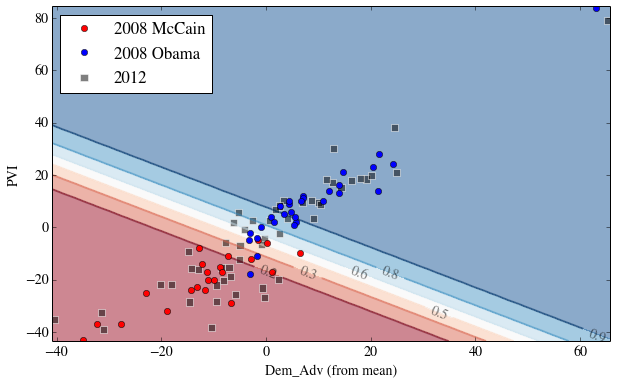Answer: The sharpness of the classifier boundary, as defined by the closeness of the contours near a probability of 0.5 gives us a sense of precision. Imagine that the boundary is very tight, tighter than what you can see in the graph. Then most states will be away from the 0.5 line, and the spread in the results will be less, or the precision higher. This is not the only consideration: indeed one must ask, how many states fall smack into the middle, say between 0.3 and 0.7. The more that do, the less precise the results will be, as there will be a greater number of simulations in which they will cross-over to another party.

To assess accuracy, we would have to see the actual outcome of the states in 2012. Accuracy would be a function of the number of states that end up on the "wrong" side of the 0.5 line, and how deep they end up on the wrong side. In terms of characterizing the 2008 outcomes, it seems that the classifier is quit eaccurate, with most misclassifications appearing in grey area of the classification boundary.

## Question 3: Trying to catch Silver: Poll Aggregation¶

In the previous section, we tried to use heterogeneous side-information to build predictions of the election outcome. In this section, we switch gears to bringing together homogeneous information about the election, by aggregating different polling result together.

This approach -- used by the professional poll analysists -- involves combining many polls about the election itself. One advantage of this approach is that it addresses the problem of bias in individual polls, a problem we found difficult to deal with in problem 1. If we assume that the polls are all attempting to estimate the same quantity, any individual biases should cancel out when averaging many polls (pollsters also try to correct for known biases). This is often a better assumption than assuming constant bias between election cycles, as we did above.

The following table aggregates many of the pre-election polls available as of October 2, 2012. We are most interested in the column "obama_spread". We will clean the data for you:

In :
multipoll = pd.read_csv('data/cleaned-state_data2012.csv', index_col=0)

#convert state abbreviation to full name
multipoll.State.replace(states_abbrev, inplace=True)

#convert dates from strings to date objects, and compute midpoint
multipoll.start_date = multipoll.start_date.apply(pd.datetools.parse)
multipoll.end_date = multipoll.end_date.apply(pd.datetools.parse)
multipoll['poll_date'] = multipoll.start_date + (multipoll.end_date - multipoll.start_date).values / 2

#compute the poll age relative to Oct 2, in days
multipoll['age_days'] = (today - multipoll['poll_date']).values / np.timedelta64(1, 'D')

#drop any rows with data from after oct 2
multipoll = multipoll[multipoll.age_days > 0]

#drop unneeded columns
multipoll = multipoll.drop(['Date', 'start_date', 'end_date', 'Spread'], axis=1)

#drop rows with missing data
multipoll.dropna()


Out:
Pollster State MoE Obama (D) Romney (R) Sample obama_spread poll_date age_days Votes
0 Rasmussen Reports Washington 4.5 52 41 500 11 2012-09-26 00:00:00 6.0 12
1 Gravis Marketing Washington 4.6 56 39 625 17 2012-09-21 12:00:00 10.5 12
2 Elway Poll Washington 5.0 53 36 405 17 2012-09-10 12:00:00 21.5 12
3 SurveyUSA Washington 4.4 54 38 524 16 2012-09-08 00:00:00 24.0 12
4 SurveyUSA Washington 4.4 54 37 524 17 2012-08-01 12:00:00 61.5 12

3.1 Using this data, compute a new data frame that averages the obama_spread for each state. Also compute the standard deviation of the obama_spread in each state, and the number of polls for each state.

Define a function state_average which returns this dataframe

Hint

pd.GroupBy could come in handy

In :
"""
Function
--------
state_average

Inputs
------
multipoll : DataFrame
The multipoll data above

Returns
-------
averages : DataFrame
A dataframe, indexed by State, with the following columns:
N: Number of polls averaged together
poll_mean: The average value for obama_spread for all polls in this state
poll_std: The standard deviation of obama_spread

Notes
-----
For states where poll_std isn't finite (because N is too small), estimate the
poll_std value as .05 * poll_mean
"""

def state_average(multipoll):
groups = multipoll.groupby('State')
n = groups.size()
std[std.isnull()] = .05 * mean[std.isnull()]
return pd.DataFrame(dict(N=n, poll_mean=mean, poll_std=std))


Lets call the function on the multipoll data frame, and join it with the electoral_votes frame.

In :
avg = state_average(multipoll).join(electoral_votes, how='outer')

Out:
State
Alabama NaN NaN NaN 9
Arizona 20 -5.500000 4.559548 11
Arkansas 3 -20.333333 4.041452 6
California 20 18.950000 5.548589 55

Some of the reddest and bluest states are not present in this data (people don't bother polling there as much). The default_missing function gives them strong Democratic/Republican advantages

In :
def default_missing(results):
red_states = ["Alabama", "Alaska", "Arkansas", "Idaho", "Wyoming"]
blue_states = ["Delaware", "District of Columbia", "Hawaii"]
results.ix[red_states, ["poll_mean"]] = -100.0
results.ix[red_states, ["poll_std"]] = 0.1
results.ix[blue_states, ["poll_mean"]] = 100.0
results.ix[blue_states, ["poll_std"]] = 0.1
default_missing(avg)

Out:
State
Alabama NaN -100.00 0.100000 9
Arizona 20 -5.50 4.559548 11
Arkansas 3 -100.00 0.100000 6
California 20 18.95 5.548589 55

#### Unweighted aggregation¶

3.2 Build an aggregated_poll_model function that takes the avg DataFrame as input, and returns a forecast DataFrame in the format you've been using to simulate elections. Assume that the probability that Obama wins a state is given by the probability that a draw from a Gaussian with $\mu=$poll_mean and $\sigma=$poll_std is positive.

In :
"""
Function
--------
aggregated_poll_model

Inputs
------
polls : DataFrame
DataFrame indexed by State, with the following columns:
poll_mean
poll_std

Returns
-------
A DataFrame indexed by State, with the following columns:
Obama: Estimated probability that Obama wins the state
"""
def aggregated_poll_model(polls):
sigma = polls.poll_std
prob =  .5 * (1 + erf(polls.poll_mean / np.sqrt(2 * sigma ** 2)))


3.3 Run 10,000 simulations with this model, and plot the results. Describe the results in a paragraph -- compare the methodology and the simulation outcome to the Gallup poll. Also plot the usual map of the probabilities

In :
#your code here
model = aggregated_poll_model(avg)
sims = simulate_election(model, 10000)
plot_simulation(sims)
plt.xlim(250, 400)

Out:
(250, 400)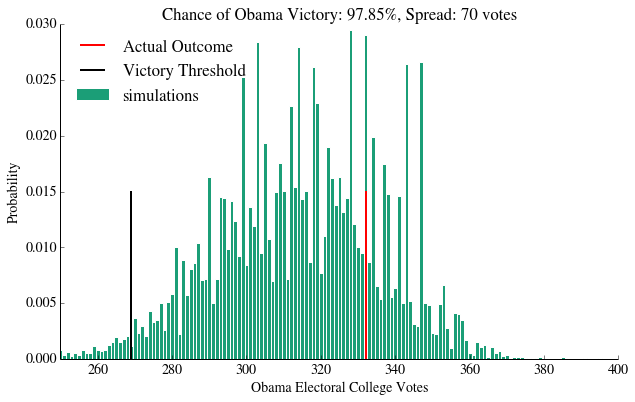Answer: The accuracy of this poll is somewhat greater than just taking the gallup poll. This is probably because

1. We're using as inputs polls that are trying to predict the election, so there is nothing "lost in translation", and
2. We are averaging many polls together, so some of their biases are likely to offset each other.

One problem is that we treated all polls as equal. Thus a bad poll with a small sample size is given equal footing as a good poll with a large one. Thus we are introducing bias both due to individual poll biases and individual poll sampling errors.

Furthermore, we estimate the standard deviation by simply taking the spread of the percentages in the polls, without considering their individual margins of error. In the limit of very limit sampling error per poll, this might be ok. However in states with some polls with large margins of error (Kansas, for eg), this can lead to an overestimate of the standard deviation, pushing the predicted probability toward 0.5. This, in turn, can hurt precision since it increases the chance that a state will flip sides in a simulation.

In :
#your code here
make_map(model.Obama, "P(Obama): Poll Aggregation")

Out:
<matplotlib.axes.AxesSubplot at 0x10de3ead0>